# Ignoring Blank Cells when Performing Calculations

While performing the calculation to ignore the blank cells we will use ISNUMBER, AND and IF formulas in Microsoft Excel.

ISNUMBER: This function can be used to check if a cell contains a number.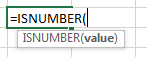For Example:-

• Cell A2 contains the number 123
•  =ISNUMBER (A2), function will return “TRUE”
• Cell A3 contains the text “XYZ”
• =ISNUMBER (A3), function will return “FALSE”

IF: -IF condition allows us to use multiple conditions in a cell. It helps us to make the function flexible and Excel allows upto a max of 64 conditions which can be checked in a cell.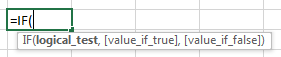Example:Cells A2 and A3 contain the numbers 3 and 5. If the cell contains 3, then the formula should display “Yes” otherwise “No”.

=IF (A1=3,”Yes”,”No”)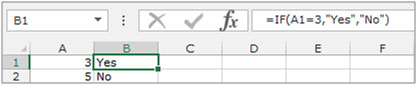AND: This function is an operator function and is used to combine multiple criteria for a cell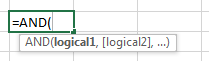Example:Column A contains 3 numbers. We need to check if these numbers are greater than 10 and less than or equal to 50.

=AND(A1>10,A1<=50)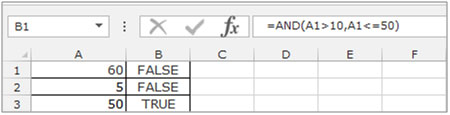2 of the cells have numbers which are not greater than 10 AND less than or equal to 50. Hence they show as False, while the last number has a True result.

Let’s take an example and understand how you can ignore blank cells when performing calculations.

We have 2 lists in columns A & B, in which some cells are containing numbers and some cells are blank. In this example we need to multiply the numbers from the corresponding cells in both the columns. Our condition is that if any cell among the two is blank, the formula should give a blank result, else it should multiply the numbers in both the cells.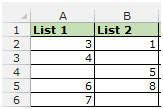• Select the cell C2.
• Enter the formula =IF(AND(ISNUMBER(A2), ISNUMBER(B2)),A2*B2,” “)
• Press enter on the keyboard.
• The function will return 3 in cell C2, as both the cells contain numbers.
• Copy the formula by pressing the key “CTRL+C” and paste it in the range C3:C6 by pressing the key “CTRL+V” on the keyboard.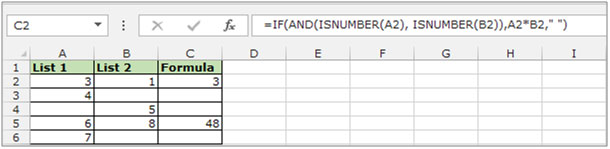We find that once the formula has been copied to the whole column, only cells C2 and C5 show the result, while C3, C4 and C6 are blank as any of the cells in these rows are blank.

In this way you can use this formula to ignore the blank cells while the calculation is performed.If you liked our blogs, share it with your friends on Facebook. And also you can follow us on Twitter and Facebook.
We would love to hear from you, do let us know how we can improve, complement or innovate our work and make it better for you. Write us at info@exceltip.com

## Users are saying about us...

1.I have a formula to calculate time as follows, where C9 is 8:00am and D9 is 6:00PM:

=IF(D9>C9,D9-C9,1-(C9-D9))

This returns a value of 10.

I want to edit the formula to return a blank cell if C9 and D9 were empty.

Help me!!??

•Hi Jenna,
if you want to return a blank cell if any of C9 and D9 is empty then write this formula.

=IF(OR(D9=””,C9=””),” “,IF(D9>C9,D9-C9,1-(C9-D9)))

if you want to return a blank cell if both C9 and D9 are empty then write this formula.

=IF(AND(D9=””,C9=””),” “,IF(D9>C9,D9-C9,1-(C9-D9)))

2.My current formula is to compare goals scored in a football game. =IF(M3=P3,1,0) is the formula I’m using to calculate a draw, how do I change this to produce a blank result if either/both M3 & P3 are blank?

•Hey Dean,

use this formula

IF(OR(M3=””,P3=””),IF(M3=P3,1,0))

3.Alfonso L Vargas Roman

Thanks for this tip,

I tried dozens of formulas unsuccessfully but your’s work. I was adding contiguous cells on each of hundred rows to then obtain an average of the sums. Some formulas returned unwanted zeros or blanks. I just wanted zeros if the sum really returned zeros or blanks if the rows were empty.

However, it caused an unwanted situation. I have an Index to identify the highest value of the sums highlighting the corresponding range row. Now I have alternated highlighted rows in the blank or empty rows (all the rows have alternated fill as a visual aid). How can I fix this thing?

4.Hi! How can i make this formula to show me the result if one or more of the cells in the formula are blank?
I have the following formula in my excel and i don’t know exactly how to make it show me the result:
=IF(AND(ISNUMBER(’31′!I8),ISNUMBER(’30′!I8),’31′!I8+’30′!I8)
it shows me only true or false because one of the cells are blank
Thank you in advance for your help

5.I am trying to formulate averages of multiple cells, but in some cells in the row there is no value. I keep getting the #DIV/0! Error. How do I make it give the average while ignoring the blank cells? Thanks!!

6.I am trying to get a cell to add one day to the date in A5 (=A5+1), but return nothing if A5 is blank. Anyone know how?

•Hi Tovah,
Use this formula
=IF(ISBLANK(A5),””,A5+1)

Terms and Conditions of use

The applications/code on this site are distributed as is and without warranties or liability. In no event shall the owner of the copyrights, or the authors of the applications/code be liable for any loss of profit, any problems or any damage resulting from the use or evaluation of the applications/code.